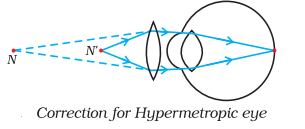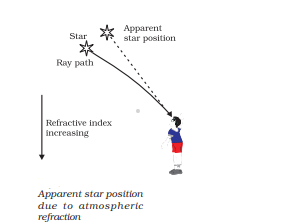# Human Eye and Colourful World Class 10 NCERT solutions

In this page we have Human Eye and Colourful World Class 10 NCERT solutions . Hope you like them and do not forget to like , social shar and comment at the end of the page.

## NCERT intext Solutions

Question 1.
What is meant by power of accommodation of the eye?
Power of Accommodation is the ability of the eye's lens to change its shape to focus on objects at different distances. The ciliary muscles control this change in shape. When viewing objects closer than the least distance of distinct vision, the eye must exert more effort to focus, which can lead to eye strain.

Question 2.
A person with a myopic eye cannot see objects beyond 1.2 m distinctly.What should be the type of the corrective lens used to restore proper vision?
Concave lens

Question 3.
What is the far point and near point of the human eye with normal vision?
Far point is infinity and near point is 25 cm

Question 4.
A student has difficulty reading the blackboard while sitting in the last row. What could be the defect the child is suffering from? How can it be corrected?
Myopia and concave lens

## NCERT Exercise Solutions

Question 1.
The human eye can focus on objects at different distances by adjusting the focal length of the eye lens. This is due to
(a) presbyopia.
(b) accommodation.
(c) near-sightedness.
(d) far-sightedness.
(b)
Question 2.
The human eye forms the image of an object at its
(a) cornea.
(b) iris.
(c) pupil.
(d) retina.
(d)
Question 3.
The least distance of distinct vision for a young adult with normal vision is about
(a) 25 m.
(b) 2.5 cm.
(c) 25 cm.
(d) 2.5 m.
(c)
Question 4.
The change in focal length of an eye lens is caused by the action of the
(a) pupil.
(b) retina.
(c) ciliary muscles.
(d) iris.
(c)
Question 5.
A person needs a lens of power –5.5 dioptres for correcting his distant vision. For correcting his near vision he needs a lens of power +1.5 dioptre. What is the focal length of the lens required for correcting (i) distant vision, and (ii) near vision?
(i) Distint vision
Power=-5.5D
Focal length = $\frac {1}{-5.5}= -.1818$ cm = $-18.18$ cm
Since it is negative, it is concave lens
(ii) Near vision
Power=1.5D
Focal length = $\frac {1}{1.5}= .666$ cm = $66$ cm
Since it is positive, it is convex lens

Question 6.
The far point of a myopic person is 80 cm in front of the eye. What is the nature and power of the lens required to correct the problem?
We know from lens formula
$\frac {1}{f}= \frac {1}{v} - {1}{u}$
The image should be formed at 80cm for any distance
$\frac {1}{f}= \frac {1}{-80} - {1}{\infty}$
$f=-80 cm$
$\text{power} = \frac {1}{f}=-1.25D$
It is concave lens

Question 7.
Make a diagram to show how hypermetropia is corrected. The near point of a hypermetropic eye is 1 m. What is the power of the lens required to correct this defect? Assume that the near point of the normal eye is 25 cm.
(a)(b)As the near point of defective eye is 1 m, the corrective lens should form the image of object at 25 cm to 1m.
We know from lens formula
$\frac {1}{f}= \frac {1}{v} - {1}{u}$
$\frac {1}{f}= \frac {1}{-100} - {1}{-25}$
or $f= 100/3$ cm or $f=1/3$ m
Power of lens = 1/f = 3D
It will be convex lens

Question 8.
Why is a normal eye not able to see clearly the objects placed closer than 25 cm?
The inability of a normal human eye to see objects placed closer than 25 cm clearly is due to the limitations in the eye's ability to accommodate or adjust its focal length. The eye lens cannot become sufficiently curved to focus the image on the retina

Question 9.
What happens to the image distance in the eye when we increase the distance of an object from the eye?
On increasing the distance of an object from eye, image distance will remain unchanged.

Question 10.The mechanism of twinkling is given below
(i)As starlight passes through the Earth's atmosphere, it is refracted multiple times due to the varying refractive indices of the different atmospheric layers.
(ii)The Earth's atmosphere is turbulent, with constant motion and mixing of air masses. This turbulence causes rapid and random changes in the refractive index along the path of the starlight.
The continuous changes in the refractive index cause the path of the starlight to bend and shift rapidly. This leads to variations in the apparent brightness and position of the star as seen from the Earth's surface.
(iii)Stars are so far away that they appear as point sources of light. Even slight changes in the path of their light can lead to noticeable fluctuations in brightness, resulting in the twinkling effect.

Question 11.
Explain why the planets do not twinkle.
The planets are much closer to the earth, and are thus seen as extended sources.If we consider a planet as a collection of a large number of point-sized sources of light, the total variation in the amount of light entering our eye from all the individual point-sized sources will average out to zero, thereby nullifying the twinkling effect

Question 12.
Why does the sky appear dark instead of blue to an astronaut?
Since there is no scattering of sunlight in space, there is no diffuse sky radiation to create the blue color that we see on Earth. The sky, therefore, appears black or dark to an astronaut.

## Summary

This Human Eye and Colourful World Class 10 NCERT solutions is prepared keeping in mind the latest syllabus of CBSE . This has been designed in a way to improve the academic performance of the students. If you find mistakes , please do provide the feedback on the mail.